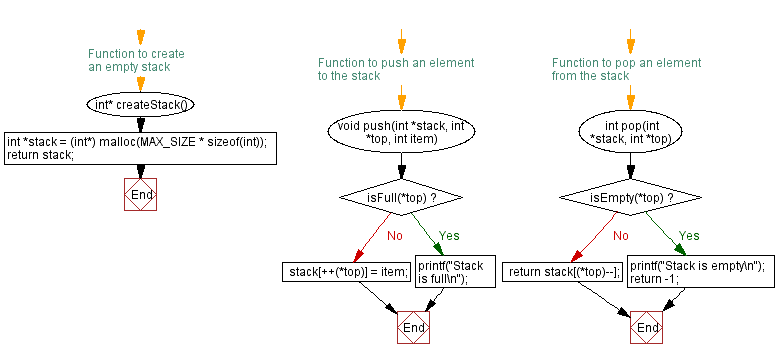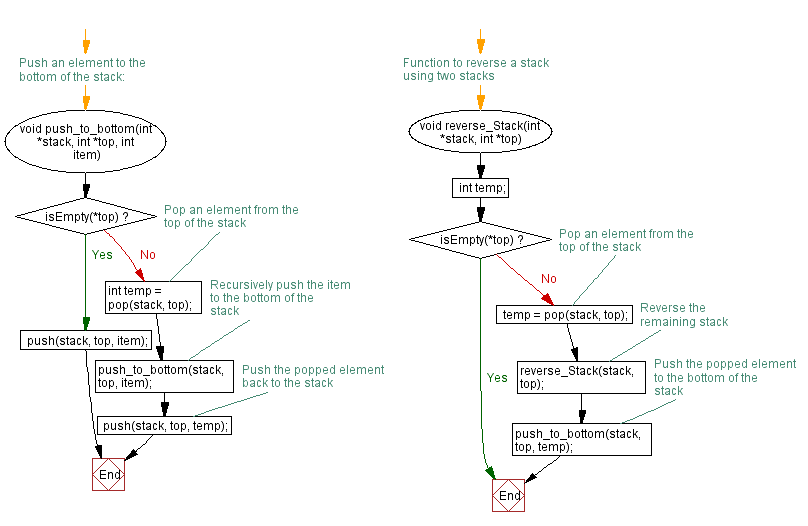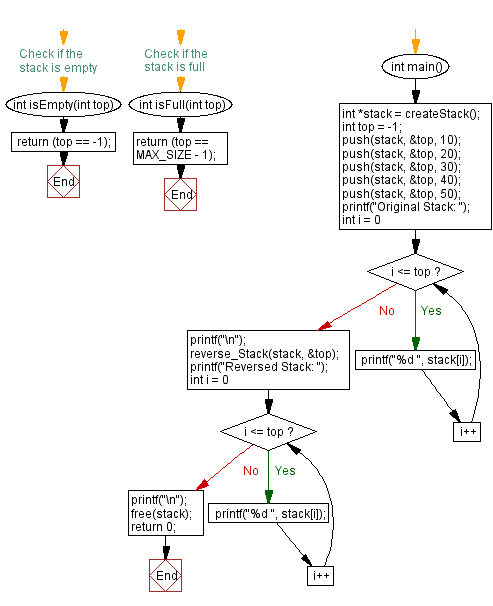﻿ C - Reverse a stack using push and pop

# C Exercises: Reverse a stack using push and pop

## C Stack: Exercise-10 with Solution

Write a C program that reverses a stack using only stack operations push and pop.

Sample Solution:

C Code:

``````#include <stdio.h>
#include <stdlib.h>

#define MAX_SIZE 100

// Function to create an empty stack
int* createStack() {
int *stack = (int*) malloc(MAX_SIZE * sizeof(int));
return stack;
}

// Check if the stack is empty
int isEmpty(int top) {
return (top == -1);
}

// Check if the stack is full
int isFull(int top) {
return (top == MAX_SIZE - 1);
}
// Function to push an element to the stack
void push(int *stack, int *top, int item) {
if (isFull(*top)) {
printf("Stack is full\n");
return;
}
stack[++(*top)] = item;
}
// Function to pop an element from the stack
int pop(int *stack, int *top) {
if (isEmpty(*top)) {
printf("Stack is empty\n");
return -1;
}
return stack[(*top)--];
}

// Push an element to the bottom of the stack:
void push_to_bottom(int *stack, int *top, int item) {
if (isEmpty(*top)) {
push(stack, top, item);
} else {
// Pop an element from the top of the stack
int temp = pop(stack, top);
// Recursively push the item to the bottom of the stack
push_to_bottom(stack, top, item);
// Push the popped element back to the stack
push(stack, top, temp);
}
}

// Function to reverse a stack using two stacks
void reverse_Stack(int *stack, int *top) {
int temp;
if (isEmpty(*top)) {
return;
} else {
// Pop an element from the top of the stack
temp = pop(stack, top);
// Reverse the remaining stack
reverse_Stack(stack, top);
// Push the popped element to the bottom of the stack
push_to_bottom(stack, top, temp);
}
}
int main() {
int *stack = createStack();
int top = -1;
push(stack, &top, 10);
push(stack, &top, 20);
push(stack, &top, 30);
push(stack, &top, 40);
push(stack, &top, 50);

printf("Original Stack: ");
for (int i = 0; i <= top; i++) {
printf("%d ", stack[i]);
}
printf("\n");

reverse_Stack(stack, &top);

printf("Reversed Stack: ");
for (int i = 0; i <= top; i++) {
printf("%d ", stack[i]);
}
printf("\n");

free(stack);
return 0;
}
```
```

Sample Output:

```Original Stack: 10 20 30 40 50
Reversed Stack: 50 40 30 20 10
```

Flowchart:C Programming Code Editor:

Have another way to solve this solution? Contribute your code (and comments) through Disqus.

What is the difficulty level of this exercise?

Test your Programming skills with w3resource's quiz.

﻿

## C Programming: Tips of the Day

C Programming - How do you pass a function as a parameter in C?

Declaration

A prototype for a function which takes a function parameter looks like the following:

```void func ( void (*f)(int) );
```

This states that the parameter f will be a pointer to a function which has a void return type and which takes a single int parameter. The following function (print) is an example of a function which could be passed to func as a parameter because it is the proper type:

```void print ( int x ) {
printf("%d\n", x);
}
```

Function Call

When calling a function with a function parameter, the value passed must be a pointer to a function. Use the function's name (without parentheses) for this:

```func(print);
```

would call func, passing the print function to it.

Function Body

As with any parameter, func can now use the parameter's name in the function body to access the value of the parameter. Let's say that func will apply the function it is passed to the numbers 0-4. Consider, first, what the loop would look like to call print directly:

```for ( int ctr = 0 ; ctr < 5 ; ctr++ ) {
print(ctr);
}
```

Since func's parameter declaration says that f is the name for a pointer to the desired function, we recall first that if f is a pointer then *f is the thing that f points to (i.e. the function print in this case). As a result, just replace every occurrence of print in the loop above with *f:

```void func ( void (*f)(int) ) {
for ( int ctr = 0 ; ctr < 5 ; ctr++ ) {
(*f)(ctr);
}
}
```

Ref : https://bit.ly/3skw9Um